#### You may also like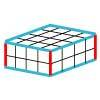### Making Cuboids

Let's say you can only use two different lengths - 2 units and 4 units. Using just these 2 lengths as the edges how many different cuboids can you make?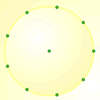How many DIFFERENT quadrilaterals can be made by joining the dots on the 8-point circle?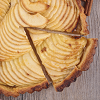### Pumpkin Pie Problem

Peter wanted to make two pies for a party. His mother had a recipe for him to use. However, she always made 80 pies at a time. Did Peter have enough ingredients to make two pumpkin pies?

# Round and Round the Circle

##### Age 7 to 11Challenge Level

I started with a clock without hands or the minute divisions (except for those where there is a number). The $12$ was replaced by a $0$ and the numbers placed outside the face.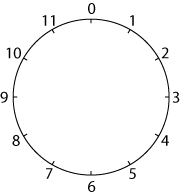I ruled lines joining up the numbers.

I started by counting in ones and I got a $12$-gon (that is a $12$-sided polygon - if you like long words you can call it a dodecagon).Then I ruled lines counting round in $2$s. And I got .....?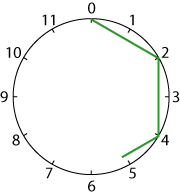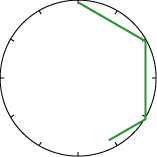Perhaps you do not need to put the numbers round the circles.

I tried $5$s (wow!) and $6$s (well!).

Each time I go on drawing lines until I get to the point where I first started.

Then I tried $7$s, $8$s, $9$s, $10$s, and $11$s.

Something interesting was happening.

Why don't you try it? What patterns do you notice emerging?

And what about counting round in $12$s?

Which shapes are the same? Can you think of a reason why?

Can you see a connection between the number in which you are counting around the circle and the number of sides in the shape you are making?